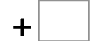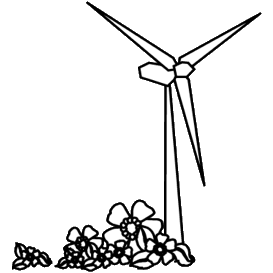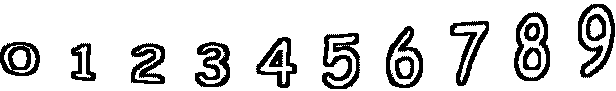20= 97   59= 10864= 125   55= 120   34= 68   53= 124 38= 104   31= 57   74= 172   81= 171